## RS Aggarwal Class 8 Solutions Chapter 12 Direct and Inverse Proportions Ex 12B

These Solutions are part of RS Aggarwal Solutions Class 8. Here we have given RS Aggarwal Solutions Class 8 Chapter 12 Direct and Inverse Proportions Ex 12B.

Other Exercises

Question 1.
Solution:
∵ x and y are inversely proportional
Then xy are equal
(i) xy = 6 x 9 = 54
= 10 x 15 = 150
= 14 x 21 = 294
= 16 x 24 = 384
∵ xy in each case is not equal
So, x and y are not inversely proportional
(ii) xy = 5 x 18 = 90
= 9 x 10 = 90
= 15 x 6 = 90
= 3 x 30 = 90
= 45 x 2 = 90
∵ xy in each case is equal
x and y are inversely proportional
(iii) xy = 9 x 4 = 36
= 3 x 12 = 36
= 6 x 9 = 54
= 36 x 1 = 36
∵ xy in each is not equal
x and y are not inversely proportional

Question 2.
Solution:
x and y are inversely proportional
xy is equal
Now,Question 3.
Solution:
Let required number of days = x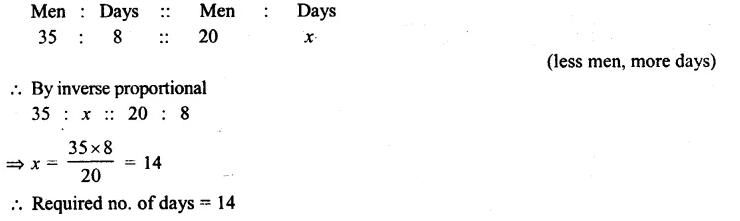Question 4.
Solution:
A pond is! dug in 8 days by = 12 men
It can be dug in 1 day by = 12 x 8 men (Less days, more men)
and it can be dug in 6 days by = $$\\ \frac { 12X8 }{ 6 }$$
= 16 men Ans. (more days, less men)

Question 5.
Solution:
Let 14 cows can graze in x days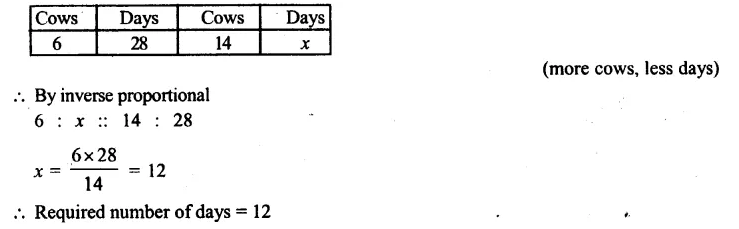Question 6.
Solution:
Let required time take = x hour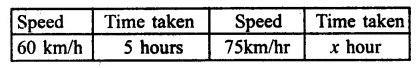By inverse proportion
60 : x :: 75 : 5
x = $$\\ \frac { 50X5 }{ 75 }$$
Time required = 4 hours

Question 7.
Solution:
Let machines required = xQuestion 8.
Solution:
Let 8 taken will fill in tank in x hourQuestion 9.
Solution:
8 taps can fill tank in = 27 minutes
1 tap can fill that tank in = 27 x 8 minutes (less tap, more time)
8 – 2 = 6 taps can fill that tank in
= $$\\ \frac { 27X8 }{ 6 }$$ minutes
= 36 minutes

Question 10.
Solution:
Let total animals can be feed with food in x days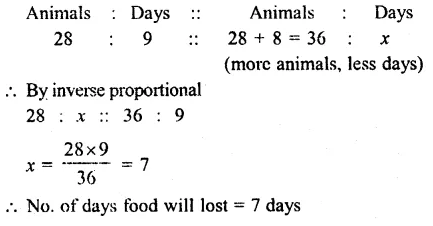Question 11.
Solution:
Let for x day, the food provision is sufficient for 900 + 500 = 1400 men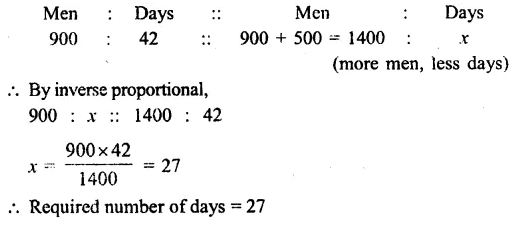Question 12.
Solution:
Let the food will be for x daysQuestion 13.
Solution:
Let each period will be of x minutes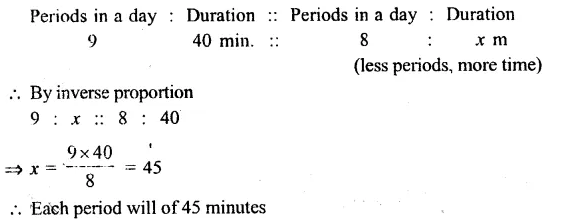Question 14.
Solution:
x and y are inversely
and x = 15, y = 6
Then xy = 15 x 6 = 90
Now if x = 9, then y = $$\\ \frac { 90 }{ 9 }$$
= 10

Question 15.
Solution:
x and y are inversely and x = 18, y = 8
xy = 18 x 8 = 144
Now if y = 16,
then x = $$\\ \frac { 144 }{ 16 }$$
= 9

Hope given RS Aggarwal Solutions Class 8 Chapter 12 Direct and Inverse Proportions Ex 12B are helpful to complete your math homework.

If you have any doubts, please comment below. Learn Insta try to provide online math tutoring for you.